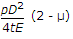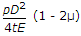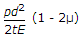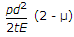# Civil Engineering - UPSC Civil Service Exam Questions

6.

When two moving bodies collide with each other, their velocity of separation bears a constant ratio to their velocity of approach. This ratio is termed as coefficient of

 A. collidity B. friction C. restitution D. permeability

Explanation:

No answer description available for this question. Let us discuss.

7.

A thin cylindrical shell of internal diameter 'D' and thickness 't' is subjected to internal pressure 'p'. The change in diameter is given by :

 A.B.C.D.Explanation:

No answer description available for this question. Let us discuss.

8.

Consider the following statements in the context of aeolian soils :
1. The soil has low density and low compressibility
2. The soil is deposited by wind
3. The soil has large permeability.
Of these statements :

 A. 1, 2 and 3 are correct B. 2 and 3 are correct C. 1 and 3 are correct D. 1 and 2 are correct

Explanation:

No answer description available for this question. Let us discuss.

9.

Which of the following pairs are correctly matched ?
1. Standard penetration test....Relative density
2. Vane shear....Cohesion
3. Consolidation test....Bearing capacity
Select the correct answer using the codes given below :

 A. 1, 2 and 3 B. 1 alone C. 1 and 2 D. 2 and 3

Explanation:

No answer description available for this question. Let us discuss.

10.

Neglecting self weight, which of the following beams will have points of contraflexure ?

 A. A simply supported beam with uniformly distributed load over part of the structure B. An overhanging beam with loading only over supported span and not on overhangs C. Fixed beam subjected to concentrated load D. Cantilever beam subjected to uniformly varying load with zero load at free end